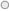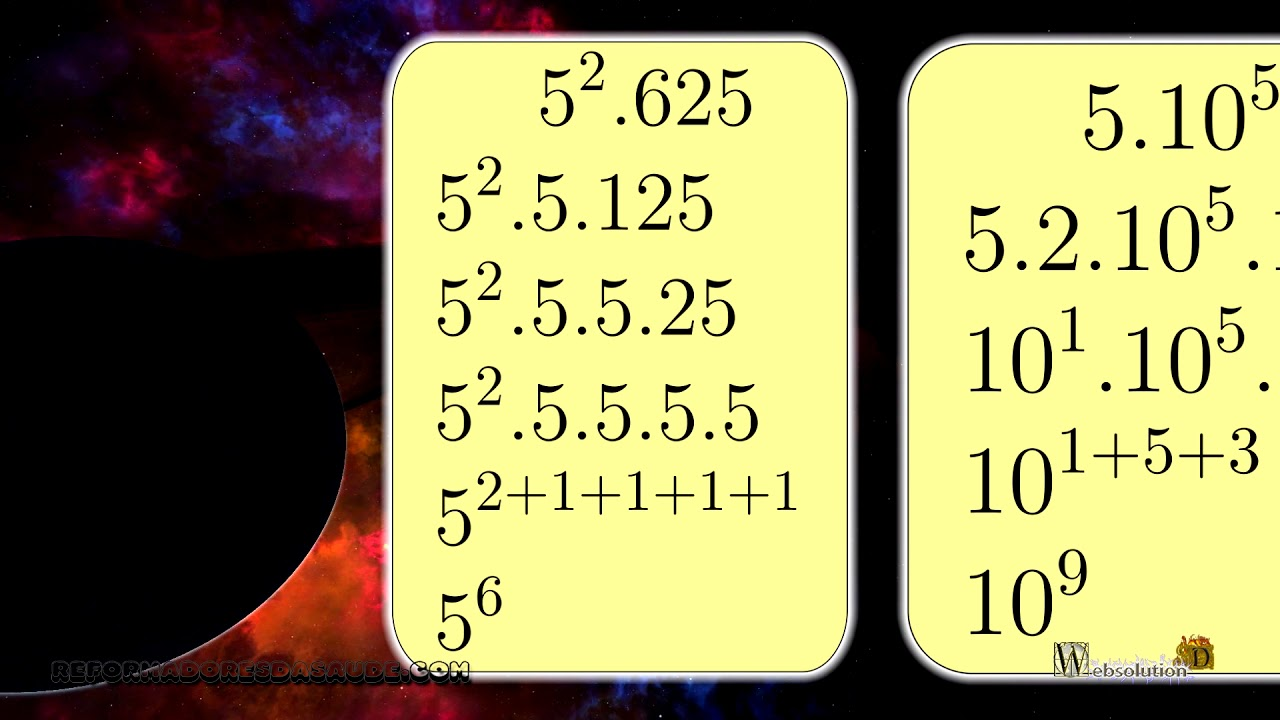## Exponentiation Exercises EC1-E1105E1-WVILet's do some potentiation exercises. Always have confidence and an open mind.
Two to fifth times 4 squared.
We have that the 2nd to the fifth cannot be simplified anymore, but the 4 can simplify, because 4 is 2 times 2. See that the 4 was squared, so the 2 raised to 1, the 1 is multiplied by the square, generating 2 squared. As they are all base 2, we can add the exponents, 5 plus 2 plus 2 that the answer is 2 raised to ninth.
3 cubed times 81, 3 cubed is already simplified, but 81 is 3 times 27, and 27 is 3 times 9, and 9 is 3 times 3.
The bases are all the same so adding up we get the value of 3 raised to the seventh power.
5 squared times 625.
5 squared is already simplified, so we have that 625 is 5 times 125, 125 is 5 times 25, and 25 is 5 times 5.
Same bases, we add and we have 5 raised to sixth power.
5 times 10 raised to fifth, 2 times 10 raised to cube.
When you see the number 5 multiplying some even number, together the two and multiply, because a value multiplied by ten will appear.
So 5 times 2 is 10, the bases are the same, so adding 10 makes us ninth power.
500 times 10 squared, 20 times 10 raised to 15. When you see numbers with zero to the right, factor out 10, so 500 is 5 times 10 times 10, and 20 is 2 times 10. Now we join the 5 with the pairs. being 5 times 2 which is ten. Now we have equal bases, just add up, which results in 10 raised to the 21st power, or ten raised to 21.
With training it is possible to make these exercises much simpler, but now we prefer to do it step by step.